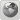Runiter Company
Would you like to react to this message? Create an account in a few clicks or log in to continue.

# Function SUM##Function SUM

Hello,

I'have just begin testing this application, which looks great. But i got a question before buying the full version :

Is it possible to draw a 3d graph with with a SUM function, like

Z(X,Y) = SUM (from i=1 to X) of F(Y)
Where X is an integer
F(Y) = 1/(1+Y)^i

If not possible, do you have any idea where i could find an application able to do that?

Thx a lot

JCAvella
Guest

##Re: Function SUM

It is certainly possible to do SUM with this softwareAre you familiar with concept of recursive calls? Smart Math Calculator supports recursive calls and SUM is a special case of a recursive call. For example the Z(X,Y) = SUM (from i=1 to X) of 1/(1+Y)^i can be represented by:

Z(X,Y)=1/(1+Y)^X+Z(X-1,Y) for as long as X is more than or equals to 1. I attached a screen shot of the complete set of expressions that address your question here:I'm also displaying the Z(3,2) which is SUM (from i=1 to 3) of 1/(1+3)^i
which is
1/(1+2)^1+1/(1+2)^2+1/(1+2)^3 = 0.4815

As you can see the program also calculates the Z(3,2) to be 0.4815

Is this what you wanted?
Also let me know if this way is too complicated. We could create a simple SUM function for the next version.

cheers,
Saeidruniter

Posts : 135
Join date : 2007-12-05
Age : 39
Location : Boston, MA, USA##Re: Function SUM

I showed you how to compute it using Smart Math Calculator, now here is the plotted graph in Graphing Calculator 3D:Let me know if this the graph that you expected.runiter

Posts : 135
Join date : 2007-12-05
Age : 39
Location : Boston, MA, USAPermissions in this forum:
You cannot reply to topics in this forum